Resistors in Series & Parallel

Before introducing the formal rules of how currents and voltages flow in circuits in general, let's look at a common scenario to try to develop intuition at a higher level.

These intuitions will be the building blocks of Kirchhoff's Voltage Law and Kirchhoff's Current Law.

The Hydraulic Analogy

Many introductory texts attempt to use a hydraulic analogy of water flowing through a pipe, with flow rate representing current and pressure representing voltage.

Caution: this analogy works at a high level, but it quickly breaks down if you look too closely or ask the wrong many questions. (Is a faucet a current source or a voltage source? What if there's air in the pipe?)

The hydraulic analogy works because conservation of charge is quite like conservation of mass in a fluid flow problem (i.e. the water has to go somewhere!), and because pressure is a scalar potential energy (per unit volume) measure just like voltage is a scalar potential energy (per unit charge).

One issue is that many people also don't have a good mechanical intuition about fluid flows, in which case the analogy doesn't add any value. Nonetheless, it's easier for most people to visualize the motion of particles they can see (i.e. water) than of invisible electrons, so the analogy adds some value. It is often reasonable for an electrical engineer to look at a schematic and think about it like a plumbing problem: where will the water (charge) flow, and where are the pressure (voltage) drops?

It is actually possible to model real fluid systems with circuits, but it is a more subtle art than you may think at first, so just take what you can without dwelling on the details too much.

If the hydraulic analogy doesn't help your intuition, don't worry about it -- you may have simply found a case where the analogy doesn't hold up.

Components in Series

Two or more components are in series if there are no side paths for current to enter or exit.

There are a pair of nodes (or terminals) that are the start and end of the chain of series elements.

Any current that goes into the first terminal must exit the second terminal.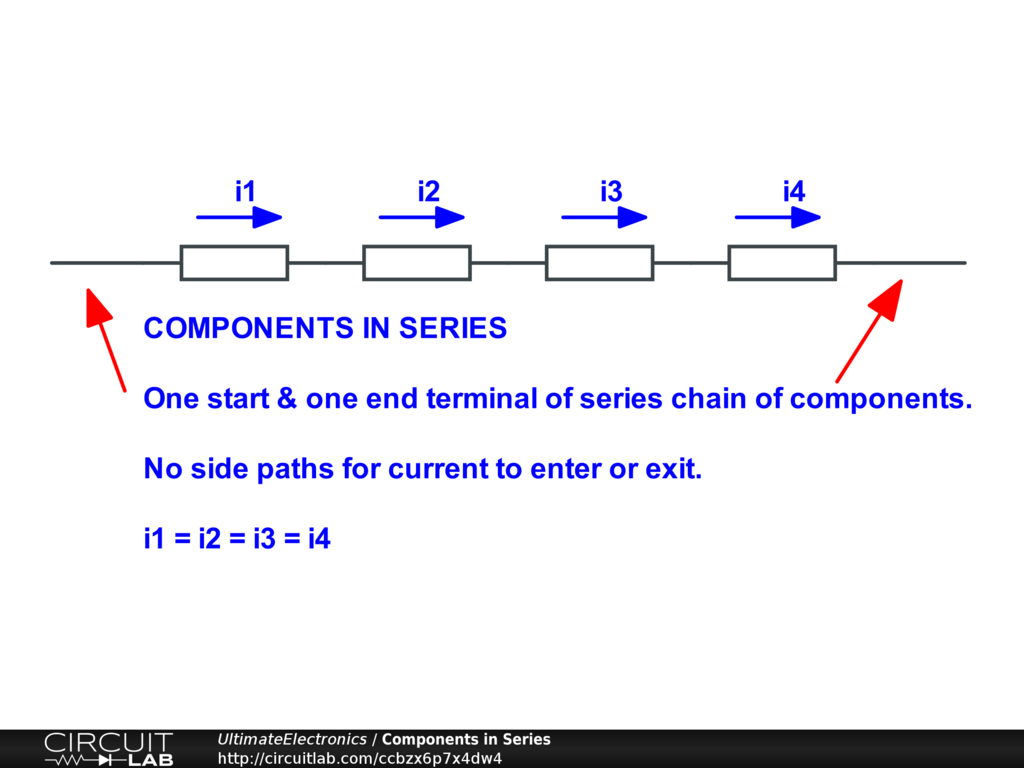For the four components drawn above, because charge is conserved:

$$i_1 = i_2 = i_3 = i_4$$

That doesn't sound so cool as an equation, but that's all that "in series" means.

In the Lumped Element Model world of schematics, this is considered to happen instantaneously. Why? Because no net charge can be stored within (or between) any circuit elements, ever. Any accumulation of charge would repel other charges and diffuse. (In reality, details like the speed of light mean this is not entirely instantaneous, but now we're talking about issues far beyond the scope of this chapter!)

In the hydraulic analogy, imagine a garden hose that's already completely filled with water. if the faucet is opened wider, the faucet pushes more water into the start of the hose, which immediately pushes on the next bit of water, and so on, until it reaches the end. The result is that even if the hose is 100 feet long, water essentially immediately starts coming out of the end because the hose was already pre-filled.

Now, if we connect a second hose to the exit of the first hose, the same current must flow through the second hose as flows through the first. We're assuming there are no leaks! But that's the point -- any possible leakage paths would have to be modeled as additional paths on the schematic, so the two hoses would no longer be simply in series.

In series means that the same flow (whether of charge or of water) must, by the layout of the system, go through all of the series components.

While the amount of water flow is the same, this does not mean the two series hoses necessarily have the same effect on the flow of water.

For example, if we have a tiny (small diameter) hose in series with a huge (large diameter) hose, the tiny hose is going to have the dominant effect on restricting the flow of water, regardless of whether it comes first or second in the direction of the flow. This may mean that, as an approximation, we may be able to ignore the huge hose and only consider the tiny hose when trying to figure out the overall flow rate; see Algebraic Approximations.

If we have two or more series components and we restrict the flow through one of them (for example, by pinching the hose), it restricts and reduces the flow rate through both of them.

In electronics, this is how an ammeter works: it's put in series with the device being measured so all the current flows through the ammeter. (A good ammeter causes very little voltage drop of its own.) However, if the resistance of the ammeter is too large, it will actually have a substantial effect on the circuit you're trying to measure -- not good!

In hydraulics, this is how a flow meter works: it's put in series so all the water flows through the flow meter. (A good flow meter causes very little pressure drop if its own.) However, if the resistance of the flow meter is too large, it will actually have a substantial effect on the flow you're trying to measure -- also not good!

Components in Parallel

Two or more components are in parallel if they are connected across the same pair of inlet and outlet nodes. (The words "inlet" and "outlet" don't really mean anything in electronics, but suggest the right idea for the hydraulic analogy.) This means that they have the same inlet voltage and outlet voltage, and therefore the same voltage difference across them.

We'll look at this schematic more closely in the next section, but for now, simply see that V1 and R2 are connected across the same two nodes: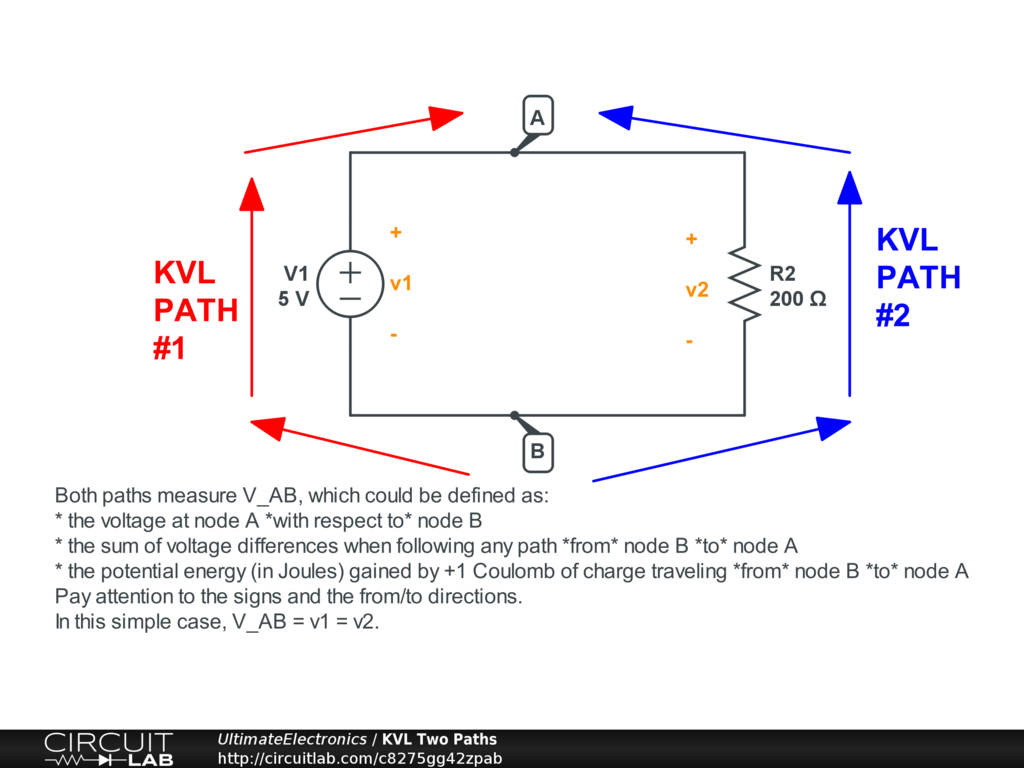Both elements are connected between nodes A and B, so:

\begin{align} v_1 & = v_2 \\ V_{A B} & = V_{A B} \end{align}

This sounds very basic as an equation, but that's all "in parallel" means.

In the world of schematics, this means that there are two (or more) paths for current to flow from point A to point B. The properties of the individual parallel branches (edges) will determine which route the current takes.

In the hydraulic analogy, imagine a splitter connected to the faucet, from which two garden hoses are connected. The pressure at the faucet-end of both is the same, and the pressure at the exit (atmospheric pressure) is the same too.

Therefore, if we open the faucet more, the additional flow will split between the two hoses.

In general, if we have two or more parallel hoses and we restrict the flow through one of them (for example, by pinching one of the hoses), it will restrict the flow through that branch, but the other branch will either unaffected -- or it may even get more flow than before (depending on the properties of the source).

In electronics, this is how a voltmeter works: it's put in parallel with the components being emasured so it measures the same voltage difference as the branch of interest experiences. However, if the resistance of the voltmeter is too small, it will actually have a substantial effect on the circuit you're trying to measure by creating an undesired leakage path -- not good!

In hydraulics, this is how a pressure gauge works: it's put in parallel (usually one side to the pressure of interest, one side to atmosphere, called "gauge pressure") so it measures the same pressure as the pipe or whatever component of interest experiences.

Resistors in Series

As discussed in the Resistance and Ohm's Law section, an individual resistor behaves as $V = I R$. So, for two resistors in series, we have: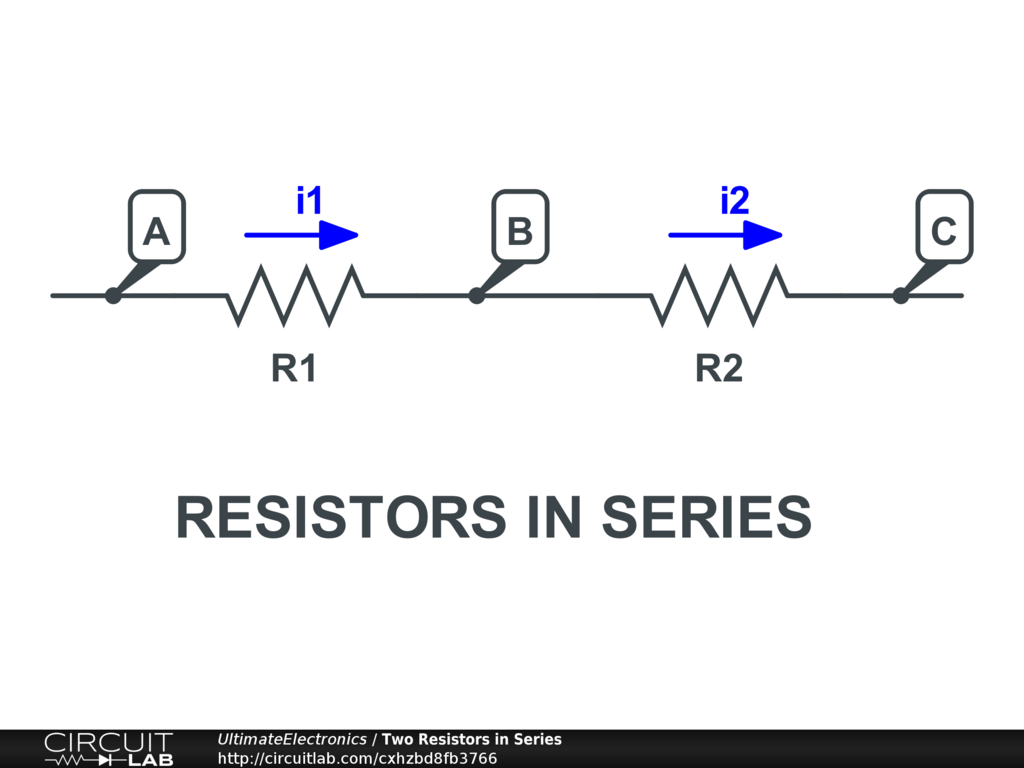\begin{align} V_{A B} & = i_1 R_1 \\ V_{B C} & = i_2 R_2 \\ \end{align}

As discussed, for any components in series, the current must be the same, so:

$$i_1 = i_2 = I$$

We can add the total voltage across both resistors:

\begin{align} V_{A C} & = V_{A B} + V_{B C} \\ & = i_1 R_1 + i_2 R_2 \\ & = I (R_1 + R_2) \end{align}

The overall series branch with two resistors behaves as though there's a single resistor with value:

$$R_{\text{series}} = R_1+R_2$$

From the external perspective of the two terminals A and C, this single resistor $R_{\text{series}}$ behaves the same (on paper) as the two individual ones in series.

Note that in reality, the single equivalent resistor may behave differently: see Practical Resistors: Power Rating (Wattage) and Practical Resistors: Temperature Coefficient. In particular, when simplifying resistors on a schematic, we often hide the fact that heat is being generated in two different components; but in reality as engineers we have to worry about each separately.

For more resistors in series, it just keeps adding:

$$R_{\text{series}} = R_1 + R_2 + R_3 + \dots$$

Resistors in Parallel

Two resistors in parallel have same voltage across both: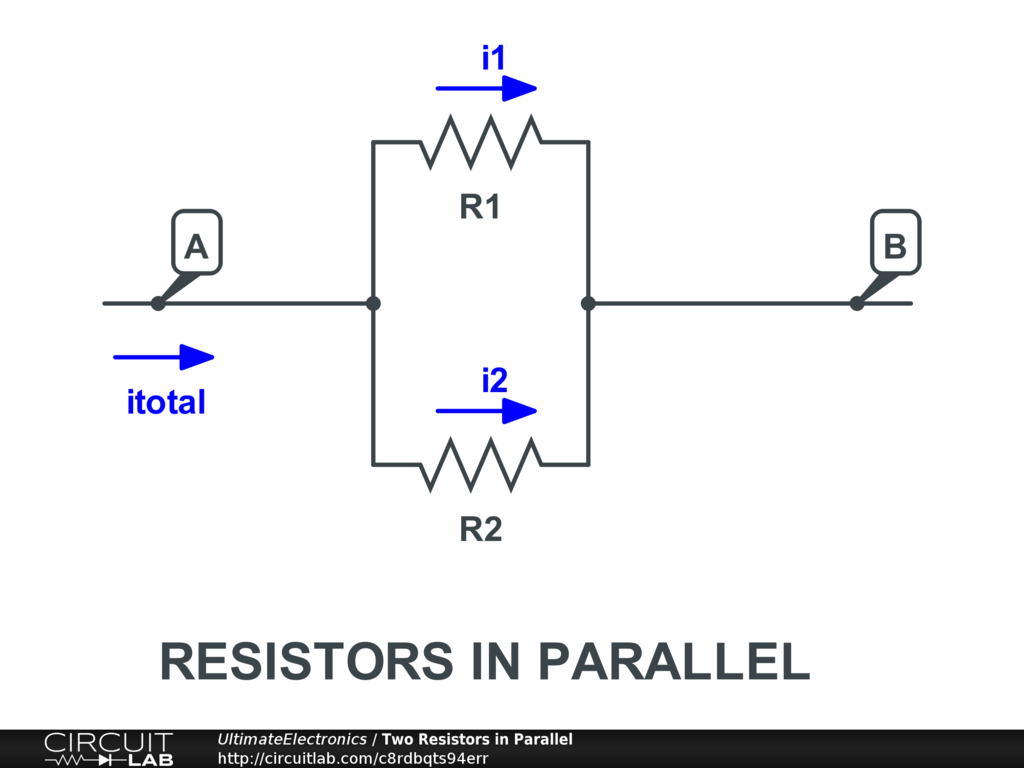$$V_{A B} = i_1 R_1 = i_2 R_2$$

Alone, there's nothing driving these two resistors.

But what if we apply an external voltage across these two resistors, or push current through them? The total current splits between the two paths:

\begin{align} i_{\text{total}} & = i_1 + i_2 \\ & = \frac {V_{A B}} {R_1} + \frac {V_{A B}} {R_2} \\ & = V_{A B} \big( \frac {1} {R_1} + \frac {1} {R_2} \big) \\ & = V_{A B} \big( \frac {R_1 + R_2} {R_1 R_2} \big) \end{align}

Since $I = \frac V R$, this is the same as a single resistor:

\begin{align} i_{\text{total}} & = V_{A B} \cdot \frac {1} {R_{\text{parallel}}} \\ \frac {1} {R_{\text{parallel}}} & = \frac {1} {R_1} + \frac {1} {R_2} \\ & = \frac {R_1 + R_2} {R_1 R_2} \end{align}

Or we can flip the fraction:

\begin{align} R_{\text{parallel}} & = \frac {1} { \frac {1} {R_1} + \frac {1} {R_2} } \\ & = \frac {R_1 R_2} {R_1 + R_2} \end{align}

This fraction with fractions in the denominator notation is a bit complicated, so to save space, sometimes electrical engineers write this fraction with two frontslashes, as:

$$R_{\text{parallel}} = \big( R_1 // R_2 \big) = \frac {1} { \frac {1} {R_1} + \frac {1} {R_2} }$$

Note that in reality, the single equivalent resistor may behave differently from the two individual ones because of heat dissipation concerns.

For multiple resistors in parallel, the current splitting rule extends:

$$R_{\text{parallel}} = R_1 // R_2 // R_3 // \dots = \frac {1} { \frac {1} {R_1} + \frac {1} {R_2} + \frac {1} {R_3} + \dots }$$

Conductance

The unit of siemens $\text{S} = \frac {1} {\Omega}$, also called "mho" (ohm spelled backwards!) is the unit of conductance. Conductance is simply the inverse of resistance:

$$G = \frac {1} {R}$$

The nice feature of conductances is that for parallel resistive paths, conductances simply add:

\begin{align} \frac {1} {R_{\text{parallel}}} & = \frac {1} {R_1} + \frac {1} {R_2} \\ G_{\text{parallel}} & = G_1 + G_2 \end{align}

Algebraic Approximations

Resistors in series and parallel provide many easy opportunities to make simplifying assumptions and approximations.

If you haven't already, review the section on Algebraic Approximations for the underlying concepts and tools needed to approach these simplifications.

For series circuits, for example, if $R_1 = 10\text{k}$ and $R_2 = 10$, then $R_{\text{series}} = R_1 + R_2 = 10010 = 10.01\text{k}$. This is so close to 10k that in many situations we can ignore the smaller resistor entirely. To formalize this rule:

$$R_{\text{series}} = R_1 + R_2 \approx R_1 \quad \text{if} \ R_1 \gg R_2$$

This matches our intuition because, since we have to "push" the same amount of charge through both an easy restriction (low resistance $R_2$) and a harder restriction (higher resistance $R_1$), it will take far more energy to push through the harder restriction, so we can virtually ignore the easier one for the sake of figuring out the total energy needed.

For parallel circuits, if $R_1 = 10\text{k}$ and $R_2 = 10$, then $R_{\text{parallel}} = \frac {R_1 R_2} {R_1 + R_2} = \frac{100000} {10010} = 9.99$, which is approximately 10. We can generalize this approximation too:

$$R_{\text{parallel}} = \frac {1} { \frac {1} {R_1} + \frac {1} {R_2} } = \frac {R_1 R_2} {R_1 + R_2} \approx R_2 \quad \text{if} \ R_1 \gg R_2$$

This matches our intuition because, since we're applying the same amount of "push" to both paths, the flow is going to mostly follow the easier restriction (low resistance $R_2$) and mostly ignore the harder restriction (higher resistance $R_1$), so the harder path only lowers the overall restriction a little bit from having the easier path alone.

Combination of Series and Parallel

You can apply these rules to simplify combinations of series and parallel circuits, but you do have to apply the rules carefully, one at a time.

For example, consider 1 resistor $R_1$ in series with a parallel pair $R_2, R_3$: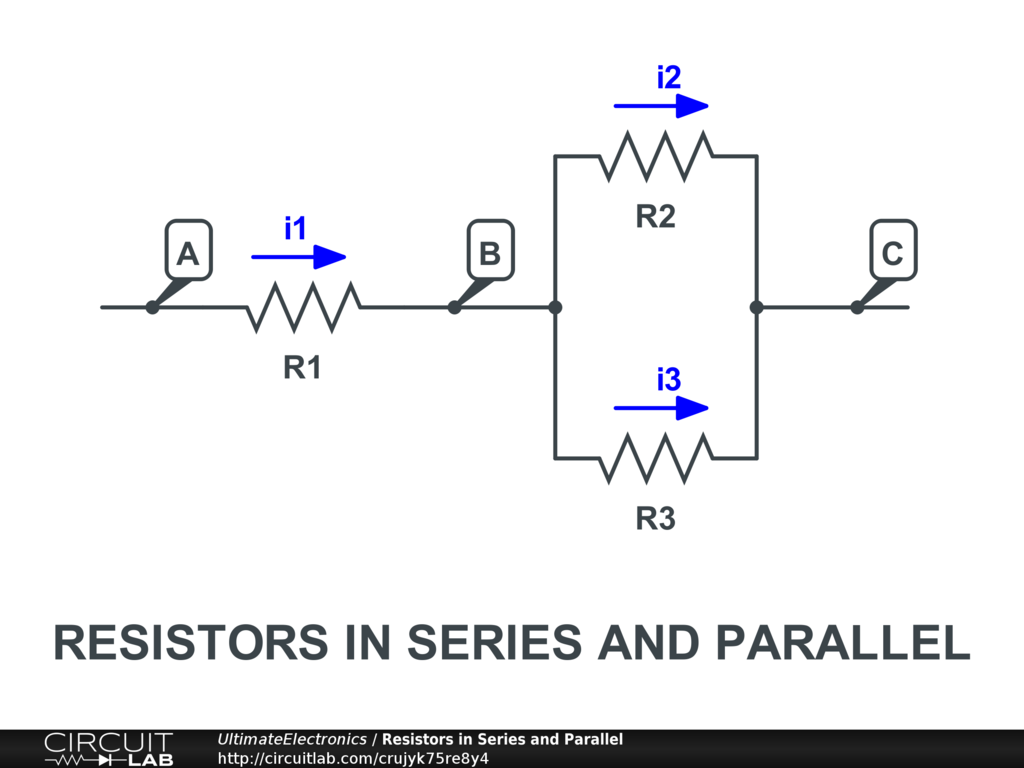The total effective resistance is:

\begin{align} R_{\text{total}} & = R_1 + \big( R_2 // R_3 \big) \\ & = R_1 + \left( \frac {1} {\frac {1} {R_2} + \frac {1} {R_3}} \right) \\ & = R_1 + \left( \frac {1} {\frac {R_2 + R_3} {R_2 R_3} } \right) \\ & = R_1 + \left( \frac {R_2 R_3} {R_2 + R_3} \right) \\ & = \frac {R_1 (R_2 + R_3) + R_2 R_3} {R_2 + R_3} \\ & = \frac {R_1 R_2 + R_1 R_3 + R_2 R_3} {R_2 + R_3} \\ \end{align}

As these expressions can quickly get complicated, it's convenient to apply the algebraic approximations discussed above where possible, or to keep them in the original, unexpanded form as long as possible for greater reading comprehension.

In other cases, it is possible to have a resistor network where you can't apply these series and parallel rules at all. For example, consider this circuit: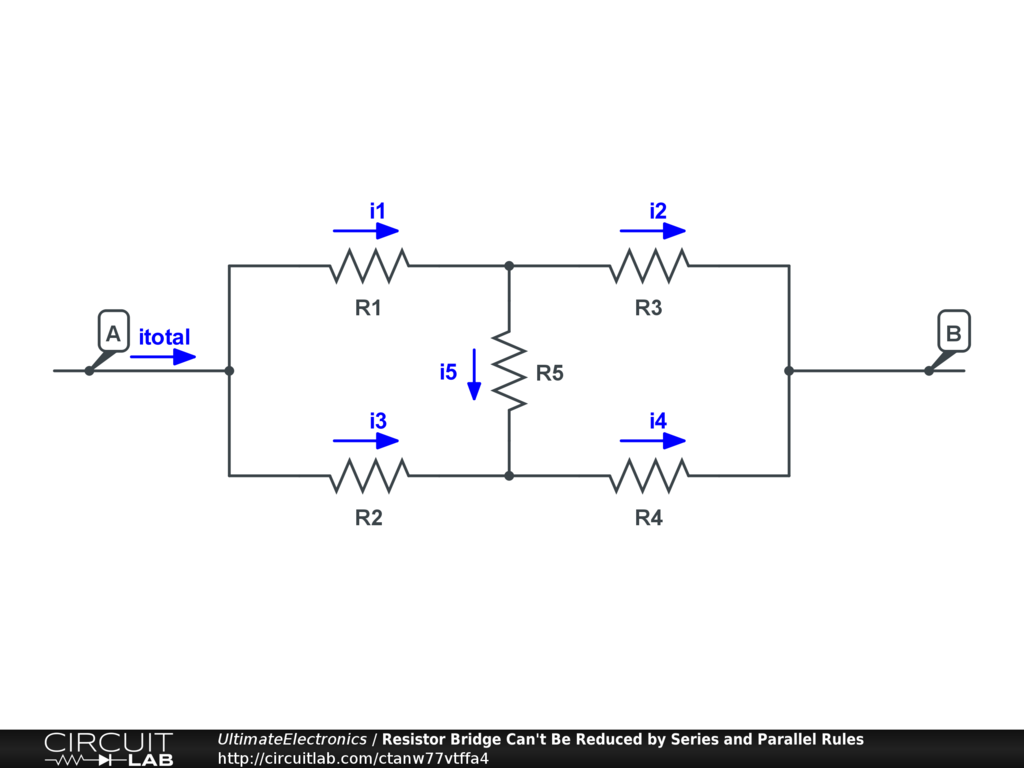This circuit has just 5 resistors, but there's nowhere to start to apply the series rule or the parallel rule.

This is not to say that it can't be reduced to a single equivalent resistance. It just can't be done through these series and parallel rules alone.

Generalization to Other Components

While we've considered the rules for resistors in this section, we want to separate which parts of our analysis are specific to resistors, and which are not.

The only fact we used about resistors is that they have a particular linear voltage-current relationship $V = I R$, which will not apply to other components.

However, there are two general rules that do apply to any components in series or parallel:

• Any two components in series carry the same current.
• Any two components in parallel have the same voltage across them.

Applying Series/Parallel Rules to Nonlinear Elements

A common question from beginners is how to simplify a circuit like this one, with a resistor in series with an LED: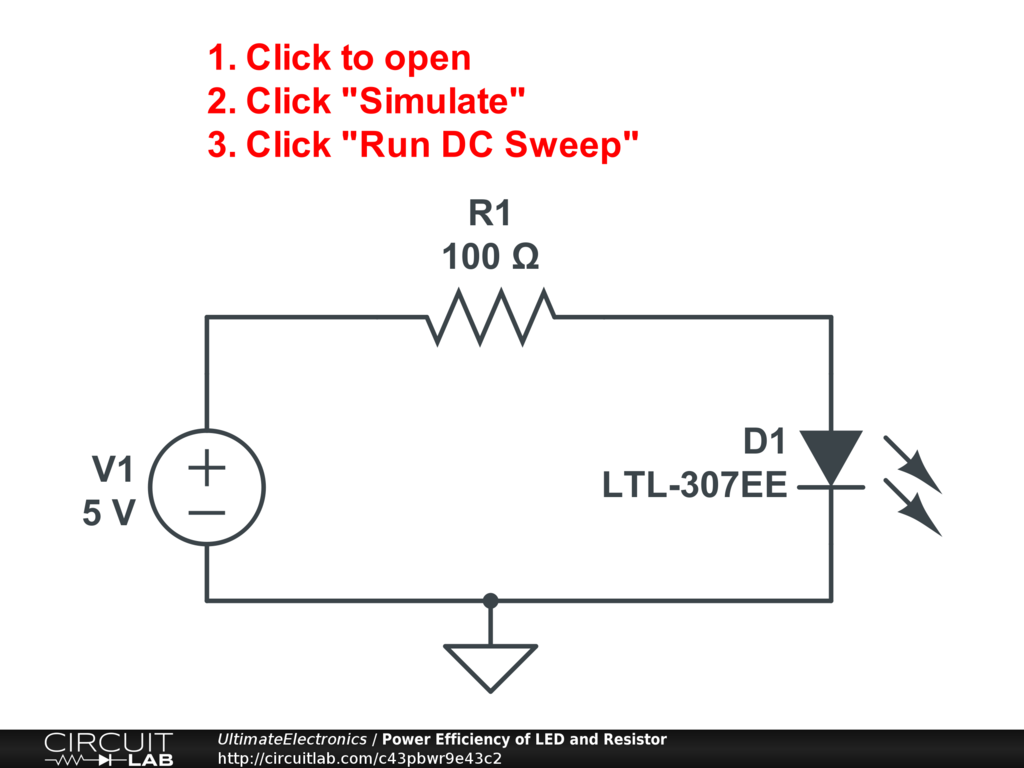The answer is that you can't combine the resistor $R_1$ with some single resistor-like value for the LED. The LED is a nonlinear component. It does not follow $V = I R$. So we can't derive a single equivalent resistance that covers all situations.

You can come up with a single equation to describe the current-voltage relationship for the resistor+LED series combination. It just won't be a linear equation!

That's why tools like circuit simulators are particularly handy: while we can reduce linear cases (like resistors in series and parallel) by hand, a simulator is capable of solving nonlinear cases, too. (See also: Linear & Nonlinear.)

What's Next

In the next section, Kirchhoff's Voltage Law and Kirchhoff's Current Law, we'll use this new intuition to develop a broader set of rules for how voltage and current behave in a circuit.